# VariableCapacitor

Ideal linear electrical capacitor with variable capacitance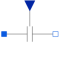# Information

This information is part of the Modelica Standard Library maintained by the Modelica Association.

The linear capacitor connects the branch voltage v with the branch current i by
i = dQ/dt with Q = C * v.
The capacitance C is given as input signal. It is required that C ≥ 0, otherwise an assertion is raised. To avoid a variable index system, C = Cmin, if 0 ≤ C < Cmin, where Cmin is a parameter with default value Modelica.Constants.eps.

Besides the Cmin parameter the capacitor model has got the two parameters IC and UIC that belong together. With the IC parameter the user can specify an initial value of the voltage over the capacitor, which is defined from positive pin p to negative pin n (v=p.v - n.v).

Hence the capacitor is charged at the beginning of the simulation. The other parameter UIC is of type Boolean. If UIC is true, the simulation tool uses

the IC value at the initial calculation by adding the equation v= IC. If UIC is false, the IC value can be used (but it does not need to!) to calculate the initial values in order to simplify the numerical algorithms of initial calculation.

# Parameters (3)

Cmin Value: Modelica.Constants.eps Type: Capacitance (F) Description: lower bound for variable capacitance Value: 0 Type: Voltage (V) Description: Initial Value Value: false Type: Boolean

# Connectors (3)

p n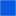Type: PositivePin Description: Positive electrical pin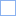Type: NegativePin Description: Negative electrical pin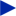Type: RealInput

# Used in Components (1)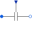VariableCapacitor Modelica.Electrical.MultiPhase.Basic Ideal linear electrical capacitors with variable capacitance